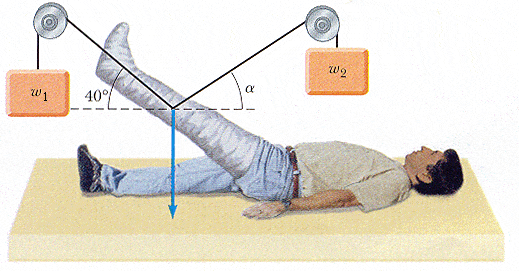# Determine the weight by using force

## Homework Statement

The leg and cast in the figure below weigh 210 N, with the center of mass as indicated by the blue arrow in the diagram. The counterbalance w1 weighs 140 N. Determine the weight w2 and the angle α needed so that no force is exerted on the hip joint by the leg plus cast.## The Attempt at a Solution

I do not even know where to begin.

## Answers and Replies

tiny-tim
Science Advisor
Homework Helper
hi mandy9008!you need to get the three forces on the leg (two tensions and the weight) to add to zero(and the tension in each string will be the same as the weight of the counterbalance)

Hello =) !

You can use the first law's Newton.

The sum of forces must be egal to 0.

P + W1 + W2 = 0

Sorry for my english level, I'm French !

Good bye !